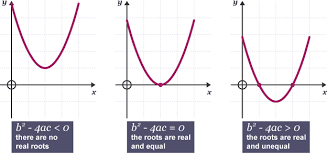# core one edexcel

• Created by: courteny
• Created on: 27-04-16 09:50

core one

1 of 9

## integrationyou add one to the power and then divided by this power

you must remember to plus c at the end of the equation

if they give you a coordinate sub that point into the equation to find c

2 of 9

## sketching graphs

f(x+a) is a translation of -a in the x direction

f(x)+a  is a translation of +a in the y direction

f(ax) is a stretch of 1/a in the x direction ( multiply x coordinates by 1/a)

af(x) is a stretch of a in the y direction ( mulitply y coordinates by a )

3 of 9

## differentiationthe gradient of the curve at a particular point is equal to the gradient of the tangent

to find a gradient of a curve dy/dx is equal to zero then sub in the x value of the point you are looking for.

find the rate of change but also subbing in the x values into dy/dx

4 of 9

## equations and inequalities

you can solve a linear equation by eliminatin or substution.

you can use substiution method to sovle simultaneous equations, where one equation is linear and the other is quadratic. you usually start by finding an expression for x or y from the linear equation.

when you multiply or divide an inequality by a negative number you need to change the inequality sign to its opposite.

to solve a quadratic inequality you solve the corresponding quadratic equation, then sketch the graph of the quadratic function then use your sketch to find the required values.

5 of 9

## laws of indices6 of 9

## co ordinate geometry

eqn of straight line = y=mx+c find gradient then sub in x and y values

parrrell lines have the same gradients

if a line has gradient m, a line perpendicular to this has a gradient -1/m

if two lines are perpendicular the product of their gradient is -1

length of a straight line=7 of 98 of 9

## arithmetic sequences and series

PROOF OF ARTHIMETIC PROGRESSION

Sn = a+(a+d)+(a+2d)......+[a+(n-2)d]+[a+(n-1)d]

Sn =[a+(n-1)d]+[a+(n-2)d]+...+(a+2d)+(a+d)+a

2Sn=[2a+(n-1)d) + [2a+(n-1)d].........

2Sn= n[2a+(n-1)d]

Sn =n/2[2a+(n-1)d]     or        n/2[a+L]            Nth term= [a+(n-1)d]

make sure you double check the years as these can be confusing

a = 1st term in sequence      d= is the difference of sequence

9 of 9